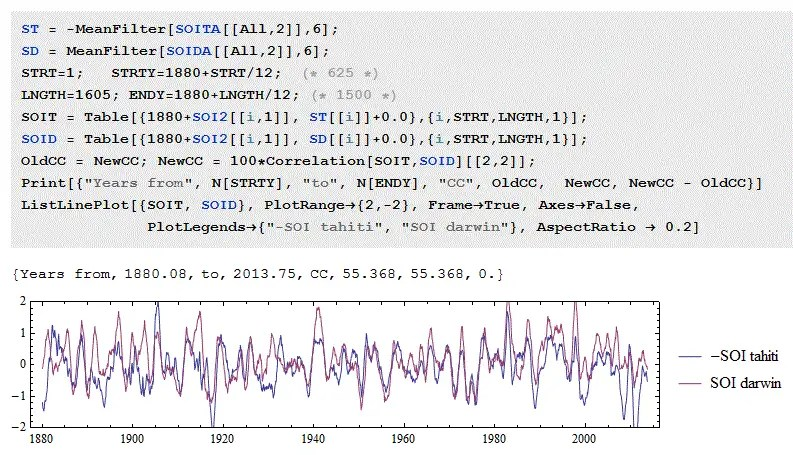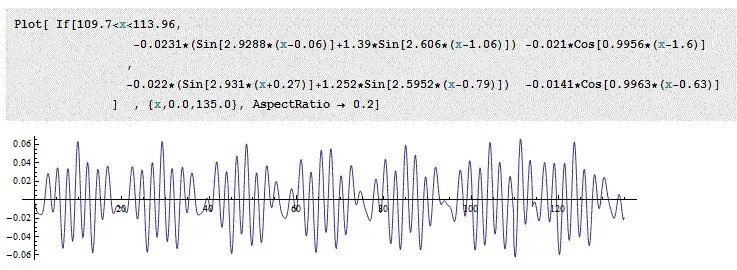# Correlation of time series

[mathjax]The Southern Oscillation embedded with the ENSO behavior is what is called a dipole , or in other vernacular, a standing wave.  Whenever the atmospheric pressure at Tahiti is high, the pressure at Darwin is low, and vice-versa.  Of course the standing wave is not perfect and far from being a classic sine wave.

To characterize the quality of the dipole, we can use a measure such as a correlation coefficient applied to the two time series.  Flipping the sign of Tahiti and applying a correlation coefficient to SOI, we get Figure 1 below:Fig 1 : Anti-correlation between Tahiti and Darwin. The sign of Tahiti is reversed to see better the correlation. The correlation coefficient is calculated to be 0.55 or 55/100.

Note that this correlation coefficient is “only” 0.55 when comparing the two time-series, yet the two sets of data are clearly aligned.  What this tells us is that other factors, such as noise in the measurements, can easily drop correlated waveforms well below unity.

This is what we have to keep in mind when evaluating correlations of data with models as we can see in the following examples.

The following is one of the best correlations I discovered when applying my sloshing model to the SOI in Figure 2 below. I was able to get the correlation coefficient to above 0.76 over the years from 1932 to 2005, by adding a modified forcing between the years 1990 to 1994 during a minimum in a beat interval (see Figure 5 here). The fit is also very sensitive to the filtering applied to the source data. Because of added noise, a more raw version of the data will result in the correlation coefficient dropping below 0.7, even though the overall fit looks as good.Fig 2: Correlation of an optimized model fit to SOI. Highlighted in yellow is the correlation coefficient multiplied by 100.

This is a view of the forcing, in Figure 2bFig 2b: Forcing function for Mathieu equation. Note the variation in beat supply between year 1880+110 and 1880+115

## SOI versus Nino 3.4

I took the Nino 3.4 anomaly from the NCDC NOAA Equatorial Pacific SST site and let Eureqa crunch on it with the Mathieu equation formulation shown below:

$$frac{d^2x(t)}{dt^2}+[a-2qcos(2omega t)]x(t)=F(t)$$

this gets transcribed as the Eureqa candidate solution:
D(x, t, 2) = f(x, t)
What Eureqa eventually finds are values for the parameters a, q cos(), and F

Of course, these need to be fed back into the differential equation and the equation solved for initial conditions to see how it matches to the actual time series.

With a Eureqa supplied 12-month smoother (sma) applied to the data before hand. The following screenshot highlights one high correlation coefficient result along the Pareto front of solutions.Fig 3:  Eureqa machine learning finds a forcing function of a period less than 1 month.

The absolutely fascinating result, which I have seen in the past with other data sets, is that it isolates periodicities of less than one calendar month, and very close to a lunar month period.

In this case, one of the sine wave components has a frequency of 83.28373 radians/year (Eureqa generates more significant digits than is displayed in the screenshot, and this extra precision is recovered by a copy&paste). That looks like unnecessary precision but watch what happens when we convert it to a period.

$$83.28373 = frac{2 pi}{T}$$

or

$$T = frac{2 pi}{83.28373} 365.242 = 27.555 days$$

As it happens, the anomalistic lunar month is 27.55455 days ! This is the average time the Moon takes to go from perigee to perigee, or the point in the Moon’s orbit when it is closest to Earth, and is a key factor in establishing the long term tidal periods.

So that is either an amazing coincidence of a random period, or this is telling us that the Nino 3.4 SST is sensitive to the anomalistic lunar month.

Yet this result is troubling as well, since the sampling period for Nino 3.4 is only a calendar month and Eureqa is picking out periods shorter than 30 days. This violates the Nyquist sampling criteria which says that sine waves would have to be at least 60 days in period to be detected. So somehow Eureqa is generating a “subsample” period that ordinarily would get aliased to a longer period. I have seen this before when applying Eureqa to the QBO data set .

This is good news but perplexing. It is good because it is all machine learning and completely hands off. All I did was apply a 12-month smoother to the data and Eureqa produced this result after a day of machine learning data crunching. I can’t see how I could have influenced the results. But how it decides to violate the Nyquist criteria is puzzling. Is it because the sampling is on the same day of the month, yet each month is of different length, and thus the data is showing subtle inflection points that Eureqa’s differential evolution algorithm is picking up? That is amazing sensitivity if that is the case.

## The Arctic Oscillation

Another dipole data set to analyze is the Arctic Oscillation. A Mathieu equation fit is shown below in Figure 4.  Based on the correlation coefficient (0.45), the fit is not very good, but because of the tight and erratic nature of the oscillations, this may be of better quality than first imagined. It all depends on whether there is a better physical model that capture the behavior more concisely.Fig 4 : Arctic Oscillation fit via Mathieu equation

## References

 Kawale, Jaya, Stefan Liess, Arjun Kumar, Michael Steinbach, Auroop R. Ganguly, Nagiza F. Samatova, Fredrick HM Semazzi, Peter K. Snyder, and Vipin Kumar. “Data Guided Discovery of Dynamic Climate Dipoles.” In CIDU, pp. 30-44. 2011. PDF link

## 5 thoughts on “Correlation of time series”

1.Keith Pickering

The Nyquist criterion is based on a uniform sampling rate, which monthly data violates because of changing month lengths. Non-uniform sampling can routinely extract signal at higher resolutions than uniform sampling, e.g., in variable star analysis.

Like

•admin

Thanks Keith, I think you are right. Eureqa doesn’t use Fourier Series techniques such as FFT which assume uniform sampling so is able to obtain a finer resolution. I suppose that one can try to align the months to a finer resolution instead of parsing the the time series into 12 equal intervals, with February keeping track of leap years.

According to the Nyquist criteria with a uniform sampling period, any algorithm should find an aliased version of this waveform, as it contains exactly the same information content. In the figure below, the sampled data points at monthly intervals intersect with the longer period aliased waveform.

As Keith suggested, What might be happening is an error in the monthly measurement in terms of time, and since the higher frequency creates a more sensitive cross-section, this period may be preferred by Eureqa.

Also possible is that because Eureqa is trying to create the lowest complexity Fourier series, it is eliminating a sine/cosine pair in favor of a single component and then is able to more easily adjust for the actual phase shift via the higher frequency waveform. In other words, it is easier to “register” the phase by sliding the higher frequency waveform than the aliased low frequency waveform.

I would also add that searching for these cycles is often maddening. To propose an attribution for an oscillation, it requires that one only identify a period and a phase. There is nothing more to it than that. Yet, this agreement could be completely coincidental. So the larger question is what does it take to make the leap from a possible coincidental agreement to something that is causal with high certainty?

The possibility that the ENSO oscillations is keyed to the long-term perigee-to-perigee lunar tidal force is extremely plausible. This is a real gravitational forcing function that could provide a definite stimulus to a sloshing behavior. After all, tides are a form of very slight sloshing of a water volume.

So the question is whether this anomalistic forcing period is emerging via a stochastic resonance with the hydrodynamic equations of the deeper water volume. That is where the physics intersects with the math, and the machine learning of Eureqa is giving us some hints of where to look.

Like

2.Will Kernkamp

Can’t wait to see results from daily sampled timeseries to confirm the finding.

Like

3.Kevin O'Neill

This same Nyquist limit question comes up a lot. Tamino covered it last year in a post simply titled, Sampling Rate. It especially seems a common misperception of those who learned their maths in electronics/engineering.

Like

4.admin

Interesting about the need for a better fit in the period from 1990 to 1994. This covers the Pinatubo volcanic event of 1991 and aftereffects.

Like